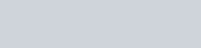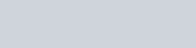How much electricity in terms of Faraday is required to produce
Question:

How much electricity in terms of Faraday is required to produce

(i) 20.0 g of Ca from molten CaCl2.

(ii) 40.0 g of Al from molten Al2O3.

Solution:

(i) According to the question,Electricity required to produce 40 g of calcium = 2 F

Therefore, electricity required to produce $20 \mathrm{~g}$ of calcium $=\frac{2 \times 20}{40} \mathrm{~F}$

= 1 F

(ii) According to the question,Electricity required to produce 27 g of Al = 3 F

Therefore, electricity required to produce $40 \mathrm{~g}$ of $\mathrm{Al}=\frac{3 \times 40}{27} \mathrm{~F}$

= 4.44 F